## Universal $L^s$-rate-optimality of $L^r$-optimal quantizers by dilatation and contraction

 Title Universal $L^s$-rate-optimality of $L^r$-optimal quantizers by dilatation and contraction Publication Type Journal Article Year of Publication 2009 Authors Abass Sagna Journal ESAIM: Probability and Statistics Volume 13 Pagination 218-246 Keywords dilatation, empirical measure theorem, Lloyd algorithm, rate-optimal quantizers Abstract We investigate in this paper the properties of some dilatations or contractions of a sequence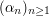of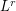-optimal quantizers of an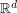-valued random vector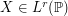defined in the probability space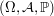with distribution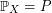. To be precise, we investigate the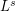-quantization rate of sequenceswhen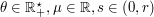orand. We show that for a wide family of distributions, one may always find parameters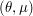such that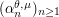is-rate-optimal. For the gaussian and the exponential distributions we show the existence of a couplesuch that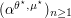also satisfies the so-called-empirical measure theorem. Our conjecture, confirmed by numerical experiments, is that such sequences are asymptotically-optimal. In both cases the sequenceis incredibly close to-optimality. However we show (see Rem. 5.4) that this last sequence is not-optimal (e.g. when,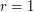) for the exponential distribution.